## Saturday, September 29, 2012

### Dimming a 12V LED strip with a mosfet and PWM, part 3

This is part 3 and for now the final part of this series of posts on my experiment with using a mosfet for dimming a 12V LED strip as the strip is going to be used for lighting up my soldering desk (undimmed).

In part two I found that using a 100nF snubber capacitor filters out the Drain-Source ringing. However I noticed a large drop in Drain voltage from the expected 12V when the mosfet is disabled. As last time, K made an interesting comment,  this time that the circuit seems seriously overdamped.

Let's try and actually use the formulas K mentions in the comments and which can be found in the article Calculating Optimal Snubbers.

The datasheet for the IRF540 mosfet shows a Drain-Source capacitance of 375pF (C) at 12V.
Assuming a ringing time (T) of 200ns and using the formula T=2*pi*sqrt(L*C) gives a parasitic inductance of 2.2uH.

This gives a snubber resistance R=sqrt(L/C) ~= 100Ω. With C=T/R this gives a snubber capacitance of 2.2nF.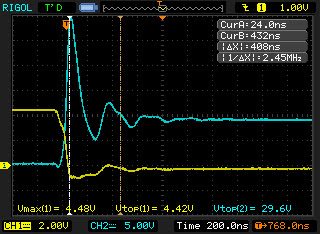100Ω 2.2nF snubber
The snubber does give a damping effect, but still a nasty initial peak of 30V.

Remeasuring the period shows actually more in the direction of 400ns. Recalculating like above gives 10uH parasitic inductance. The snubber gives 190Ω and 1nF.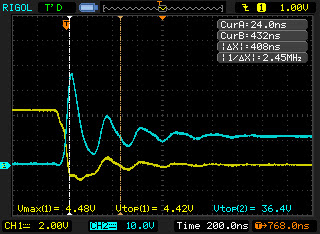190Ω 1nF snubber
Note that I changed the vertical scale of the blue line to 10V. This means we have a nasty spike of 36V, which is definitely worse.

As this still doesn't look very well I started playing with values.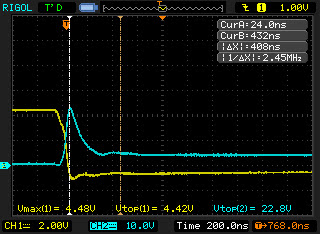47Ω 1nF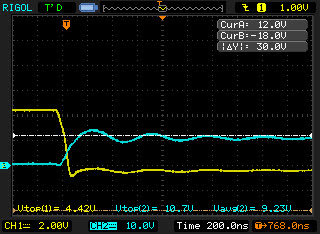0Ω 4.7nF snubber (blue 10V scale)0Ω 4.7nF snubber (blue 5V scale)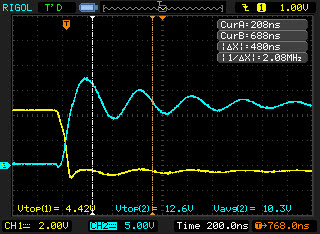0Ω 3.3nF snubber0Ω 10nF snubber
As you can see increasing the capacitance reduces the peak, but also reduces the end voltage below the expected 12V.

I finally settled for the 0Ω 3.3nF snubber. It gives some minimal ringing but stays near 12V. As it requires no resistor there is no resistive loss.

Measuring the power usage shows no significant difference between the snubbed and plain circuit. I still think that some parts of the story have not been revealed here. Maybe in a future blog post...